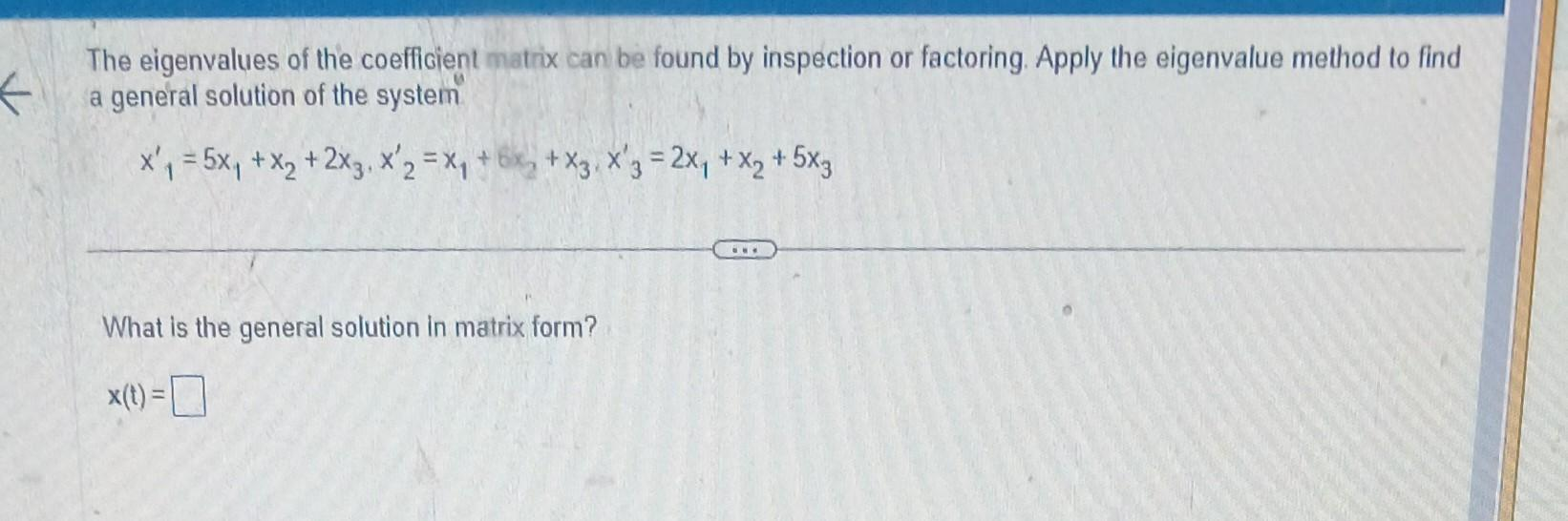# (Solved): The eigenvalues of the coefficient matrix can be found by inspection or factoring. Apply the ei ...The eigenvalues of the coefficient matrix can be found by inspection or factoring. Apply the eigenvalue method to find a general solution of the system What is the general solution in matrix form?

We have an Answer from Expert